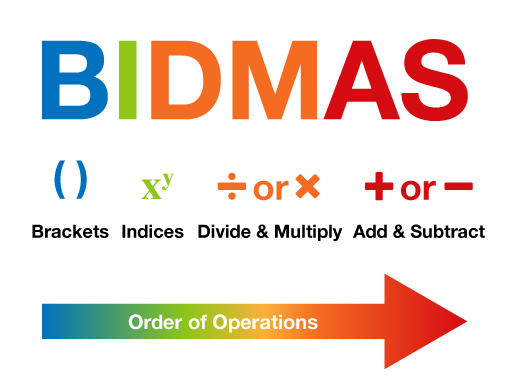Everyday maths 2 (Wales)

Start this free course now. Just create an account and sign in. Enrol and complete the course for a free statement of participation or digital badge if available.

Free course

# 11.1 Order of operations

The order in which you carry out operations can make a big difference to the final answer. When doing any calculation that involves doing more than one operation, you must follow the rules of BIDMAS in order to arrive at the correct answer.Figure 29 The BIDMAS order of operations

## B: Brackets

Any calculation that is in brackets must be done first.

Example:

2 × (3 + 5)

2 × 8 = 16

Note that this could also be written as 2 (3 + 5) because if a number is next to a bracket, it means you need to multiply.

If there is more than 1 operation in the brackets, you must follow the rules of BIDMAS in the brackets.

## I: Indices

After any calculations in brackets have been done, you must deal with any calculations involving indices or powers i.e.

32 = 3 × 3

or

43 = 4 × 4 × 4

Example:

3 × 42

3 × (4 × 4)

3 × 16 = 48

## D: Divide

Next come any division or multiplication calculations. Of these two calculations, you should do them in the order that they appear in the sum from left to right.

Example:

16 − 10 ÷ 5

16 − 2 = 14

## M: Multiply

Example:

5 + 6 × 2

5 + 12 = 17

Finally, any calculations involving addition or subtraction are done. Again, these should be done in the order that they appear from left to right.

Example:

24 + 10 − 2

34 − 2 = 32

or

24 + 8 = 32

## Activity 30: Using BIDMAS

Now have a go at carrying out the following calculations yourself. Make sure you apply BIDMAS!

1. 4 + 3 × 2
2. 5 (4 − 1)
3. 36 ÷ 32
4. 7 + 15 ÷ 3 − 4

1. 4 + 6 = 10
2. 5 × 3 = 15
3. 36 ÷ 9 = 4
4. 7 + 5 − 4 = 8

Now that you have learned the rules of BIDMAS you are ready to apply them when using formulas.

FSM_2_CYMRU#### Find out more

*Eligibility rules apply for financial support.